research papersJOURNAL OFSYNCHROTRONRADIATION
ISSN: 1600-5775
Volume 7| Part 4| July 2000| Pages 209-214

Effect of surface roughness on the spatial coherence of X-ray beams from third-generation synchrotron radiation sources

aShanghai Institute of Nuclear Research, Academia Sinica, PO Box 800-204, Shanghai 201800, People's Republic of China
*Correspondence e-mail: xiaotq@ssrc.ac.cn

(Received 13 September 1999; accepted 16 March 2000)

The effect of the surface roughness of optical elements, such as Be windows and reflection mirrors, in synchrotron radiation beamlines on the spatial coherence of the X-ray beam is investigated systematically by means of digital simulation, in which a new model for X-ray reflection from a rough surface is proposed. A universal factor is employed to evaluate the spatial coherence quantitatively, based on which critical values for surface roughness are reached. The results from simulation are consistent with those from experiments.

1. Introduction

Owing to the improvement of electron beam emittance and employment of insertion devices, the spatial coherence of X-ray beams from third-generation synchrotron radiation light sources has been improved to a great extent, making many types of coherent imaging possible, such as phase contrast, holography and microtomography.

On the other hand, various optical elements in beamlines, such as Be windows, mirrors and multilayers, have been found to exert a great influence on the spatial coherence due to their surface roughness, which introduces random and unwanted phase modulation into the X-ray beam.

Some experimental results have been obtained at ESRF (Snigirev, 1996; Snigirev et al., 1996). However, it is useful if a critical value of the surface roughness is predicted, below which the effect of roughness on the spatial coherence of the X-ray beam is acceptable. A beamline for coherent microscopy is to be built at Shanghai Synchrotron Radiation Facility (SSRF), also a third-generation light source. We have performed a systematic investigation of this issue by means of digital simulation, discussed in this paper. Firstly, in-line holograms of a single fibre are employed to evaluate the spatial coherence. Secondly, the case of Be windows for hard X-ray beamlines is investigated. Thirdly, a simple model for X-ray reflection is proposed, based on investigations with both soft and hard X-rays. Then a factor is proposed for evaluating the effect of roughness on the spatial coherence of the beam quantitatively. Finally, a comparison with experimental results is given.

2. Theories

2.1. Approach for spatial coherence evaluation

On the characterization of spatial coherence, classical techniques with two-wave interference cannot work well in the high photon energy region due to the high transmissivity of X-rays (Snigirev, 1996). Therefore, we would like to use a simple holographic technique, in-line holography, where a single fibre (such as a boron fibre) is employed, on account that holographic interference patterns are closely related to the spatial coherence of the X-ray beam. Fig. 1shows a schematic set-up for in-line holography.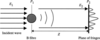Figure 1 Schematic set-up for in-line holography.

The process of in-line holography can be described by Fresnel diffraction. Assume E1 is the incident X-ray electric scalar and E2 is the electric scalar on the plane of the fringe patterns. The boron fibre is in the plane P1 which is perpendicular to the incident direction. P2 is the fringe pattern plane, Z is the distance between P1 and P2. For simplicity and clarity, we consider the incident wave to be a monochromatic planar wave which is purely coherent. According to the Fresnel diffraction equation (Goodman, 1968),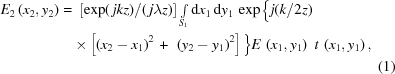where (x1, y1) and (x2, y2) are coordinates on planes P1 and P2, respectively, and t(x1, y1) is the X-ray transmissivity function in P1,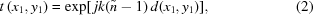whereis the complex refractive index and d(x1, y1) refers to the thickness on the plane. Assume that the boron fibre has a radius R, then the thickness on the boron fibre is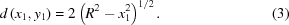The intensity I(x2, y2) on the plane P2 is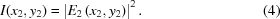2.2. Physics model

Here we would like to consider two commonly used optical elements, the Be window and the planar reflection mirror.

2.2.1. Be window

Fig. 2shows the schematic set-up of in-line holography in the case of the Be window. Here, the incident wave is a monochromatic plane wave,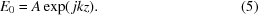After propagation through the Be window, the electric scalar changes into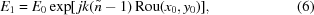where Rou(x0, y0) denotes the roughness on the Be window. After the beam is diffracted by the boron fibre, we can obtain the electric scalar in P2,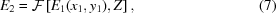where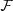represents Fresnel transformation.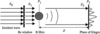Figure 2 Schematic set-up for in-line holography for the case of the Be window.
2.2.2. Mirror

Fig. 3(a) shows the schematic set-up of in-line holography in the case of the mirror. Fig. 3(b) shows a simple model for phase modulation in the X-ray beam owing to the surface roughness of mirrors. Here we ignore the effect of back-reflection and absorption, for they have no effect on the spatial coherence of the X-ray beam. The dashed line indicates the path of the X-ray reflection without roughness. Assume that the roughness at point O is Rou, then, according to the geometric relation, the optical path difference between cases with and without roughness is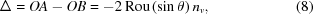where θ is the incident angle and nv is the refractive index of a vacuum. After modulation by the roughness of the mirror, the electric scalar becomes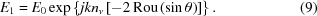After diffraction by a boron fibre, the electric scalar of each point on the fringes plane P2 can be obtained according to (7)and (4).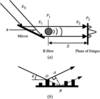Figure 3 (a) Schematic set-up for in-line holography for the case of the mirror. (b) A simple model for phase modulation of the X-ray beam by the surface roughness of the mirror.

3. Simulation

Since roughness is distributed randomly, we can use the random function to simulate the surface roughness of the optical element. Here the type of roughness is r.m.s.

We can realize the process of Fresnel diffraction through a digital two-dimensional fast Fourier transform.

In the simulation, the dimensions of the object plane P1 are set to be 100 µm × 100 µm, and the size of the image plane P2 is also 100 µm × 100 µm, so the distance between P1 and P2 can be decided accordingly. The radius of the boron fibre is 1 µm.

The sampling lattice of the object plane and the image plane is 1024 × 1024. Owing to the display model of the computer, we can only plot the fringes pattern within an area of 640 × 480 pixels.

4. Results and analysis

For Be windows, we have only investigated the case for hard X-rays, in which wavelengths of 1.0 Å (12 keV) and 0.41 Å (30 keV) are selected. As shown in Fig. 4, when roughness Rou < 0.1 µm, the contrast of the fringes pattern (CFP) changes slightly with Rou. When Rou = 0.2 µm, the change of CFP is obvious. Where Rou > 0.2 µm, CFP gradually worsens with the increment of Rou. When Rou = 5.0 µm, hardly any fringe can be observed. Fig. 5is almost the same as Fig. 4except that the wavelength is much shorter.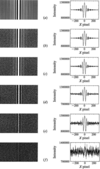Figure 4 Fringes patterns and their transverse profiles of intensity at 1.0 Å (12 keV) and different surface roughnesses of the Be window: (a) 0 µm, (b) 0.1 µm, (c) 0.2 µm, (d) 0.5 µm, (e) 1.0 µm, (f) 5.0 µm.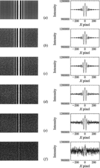Figure 5 Fringes patterns and their transverse profiles of intensity at 0.41 Å (30 keV) and different surface roughnesses of the Be window: (a) 0 µm, (b) 0.1 µm, (c) 0.2 µm, (d) 0.5 µm, (e) 1.0 µm, (f) 5.0 µm.

For plane reflection mirrors, the cases for hard X-rays (0.41 Å) and soft X-rays (43.7 Å), which is near the water window, are investigated. The film material is Au and the incident angle is a little less than the critical incident angle.

Fig. 6shows the case for hard X-rays. For a mirror at λ = 0.41 Å and θ = 2 mrad, when Rou < 0.1 Å, CFP has almost no change; when Rou > 0.2 Å, CFP gradually worsens; when Rou = 5 Å, no fringe can be seen. This implies that in the case for hard X-rays the acceptable roughness for mirrors should be less than 0.2 Å.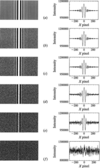Figure 6 Fringes patterns and their transverse profiles of intensity at a wavelength of 0.41 Å (30 keV), with a grazing-incident angle of 2 × 10−3 rad and different surface roughnesses of the mirror: (a) 0 Å, (b) 0.1 Å, (c) 0.2 Å, (d) 0.5 Å, (e) 1 Å, (f) 5 Å.

Fig. 7shows the case for soft X-rays. We find that for a mirror at λ = 43.7 Å and θ = 0.1 rad, the CFP does not change much until Rou > 5 Å. When Rou = 20 Å, no fringe can be observed. Therefore, the acceptable roughness of the mirror for soft X-rays should be less than 5 Å.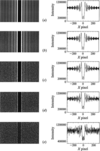Figure 7 Fringes patterns and their transverse profiles of intensity at a wavelength of 43.7 Å, with a grazing-incident angle of 0.1 rad, and different surface roughnesses of the mirror: (a) 0 Å, (b) 1 Å, (c) 5 Å, (d) 10 Å, (e) 20 Å.

All the above results are based on qualitative analysis. To evaluate the effect of roughness on spatial coherence quantitatively, a factor dr.m.s. is introduced,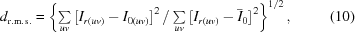where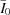represents the intensity average in the image plane, I0(uv) denotes the intensity of each point in the image plane with zero roughness, while Ir(uv) represents the intensity with roughness r introduced. dr.m.s. denotes the unified r.m.s. image distance between the image in the case of zero roughness and that in the case of r roughness. In this way the effect of roughness on the spatial coherence can be measured quantitatively by a universal criteria, and accordingly the critical value of the surface roughness, which is the maximum endurable value to guarantee good coherence of the beam, can be obtained.

Table 1gives the values of dr.m.s. for different wavelengths and roughness. The column in bold face shows the estimated critical values for roughness and image distance. Fig. 8shows their fitted curves.

 Table 1Unified r.m.s. image distance for different wavelengths and roughnesses
 Be window Rou (µm) 0.1 0.2 0.5 1.0 5.0 1.0 Å dr.m.s. 0.044 0.084 0.201 0.392 0.981 Be window Rou (µm) 0.1 0.2 0.5 1.0 5.0 0.41 Å dr.m.s. 0.032 0.062 0.149 0.295 0.838 Mirror Rou (Å) 0.1 0.2 0.5 1.0 5.0 0.41 Å dr.m.s. 0.034 0.064 0.158 0.312 0.894 Mirror Rou (Å) 1.0 5.0 10 20 43.7 Å dr.m.s. 0.009 0.052 0.155 0.609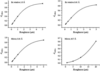Figure 8 Fitted dr.m.s. curves for different wavelengths and different roughnesses.

Combined with the analysis above, it is found that with the increment of roughness, dr.m.s. also increases. For Be windows at a wavelength of 1.0 Å, when dr.m.s. is more than 0.084, the fringes pattern becomes worse very sharply. For a Be window at 0.41 Å, when dr.m.s. is beyond 0.062, the CFP quickly worsens. The same values for mirrors are 0.064 (0.41 Å) and 0.052 (43.7 Å). Thus, more strictly, we choose the critical dr.m.s. value as 0.050, which can guarantee quite a good CFP. According to Fig. 8, the corresponding critical roughness values are 0.13 µm (Be window, at 1.0 Å), 0.15 µm (Be window, at 0.41 Å), 0.15 Å (mirror, at 0.41 Å) and 4.9 Å (mirror, at 43.7 Å). These roughness values are called maximum endurable roughness limitations, i.e. the values to guarantee a good spatial coherence of the beam.

5. Comparison with experimental results

Fig. 9shows the fringes pattern and its transverse profile of intensity at a wavelength of 1.24 Å and grazing-incident angle of 2.79 mrad (0.16°) with a surface roughness of the mirror of 3 Å (r.m.s.). We find that it is consistent with the experimental results (Snigirev, 1996), which in turn confirms the validity of our X-ray reflection model for mirrors.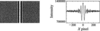Figure 9 Fringes pattern and its transverse profile of intensity with the surface roughness of the mirror of 0.3 nm, a wavelength of 1.24 Å and a grazing-incidence angle of 2.79 mrad (0.16°).

6. Discussion

The effective roughness for mirrors, Roueff = Rou(sinθ), is much less than that for Be windows, owing to the grazing incidence of the X-ray beam. However, comparing the case of Be windows with that of mirrors, we find that in the case of Be windows the spatial coherence of the X-ray beam begins to worsen gradually when Rou = 0.2 µm, while for mirrors this occurs when Rou = 0.2 Å. That is, the spatial coherence in the case of mirrors is more sensitive to surface roughness than in the case of Be windows. In fact, the phase modulation (i.e. the optical path difference) of the X-ray beam is related not only to the effective roughness but also to the complex refractive index of the material. For Be windows, the phase shift ΔδBe (the refractive index decrement of the Be window), while for mirrors Δnv (the refractive index in a vacuum). As we know, δBe/nv < 10−5. Therefore, the spatial coherence of the X-ray beam in the case of mirrors is more susceptible to surface roughness than that of Be windows.

For mirrors, only those with a plane surface are taken into account. For the case of curved surfaces the results should be similar. For ordinary shaped mirrors, e.g. plane, spherical etc., a highly smooth finish can be obtained, whereas shaped mirrors, e.g. ellipsoid, toroid etc., are difficult to polish to achieve a small roughness, especially the larger ones. Even though the current technique advances have made it possible to polish shaped mirrors to almost the same degree of finish as plane mirrors, in general the figure error is more troublesome. Therefore, in the beamline, where there are strict demands on the spatial coherence, it is advisable not to use specially shaped optical elements.

As for reflection optical elements, for hard X-rays the maximum endurable surface roughness is 0.2 Å, whereas for soft X-rays it is 5 Å. As far as current fabrication ability and checking technology is concerned, the minimum roughness within reach is about 2 Å. Thus, for soft X-ray beamlines, coherent illumination and coherent imaging are feasible while, for hard X-ray beamlines, some types of experiments such as holography are difficult to achieve owing to serious degradation of the X-ray spatial coherence by optical elements while the light beam is transmitted to experimental stations.

7. Conclusions

The third-generation synchrotron radiation light sources have quite a good spatial coherence, but this is prone to be spoiled by phase modulation due to surface roughness of the optical elements. Thus strict demands are made on the fabrication of optical elements. That is, only if the surface roughness is smaller than a critical value can a good spatial coherence of the X-ray beams be guaranteed, and as a result most of the coherent flux can remain.

Based on the analysis above, we can conclude that (a) for Be windows the surface roughness must be less than 0.15 µm, (b) for mirrors the maximum endurable surface roughness is 4.9 Å and 0.15 Å for soft X-rays and hard X-rays, respectively, in order to preserve the good spatial coherence and the high coherent flux in the X-ray beam from third-generation synchrotron radiation sources.

ReferencesJOURNAL OFSYNCHROTRONRADIATION